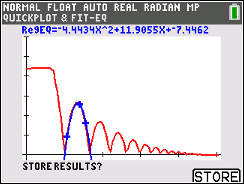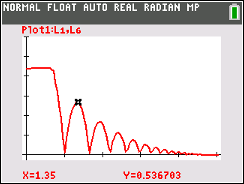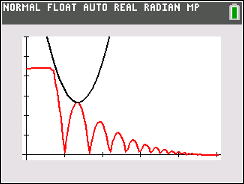••• ##### Device
• TI-83 Plus Family
• TI-84 Plus
• TI-84 Plus Silver Edition
•TI-84 Plus C Silver Edition
•TI-84 Plus CE
•TI-84 Plus CE Python
• ##### Software

TI Connect™
TI Connect™ CE

# Science: Curve Ball

by Texas Instruments#### Overview

In this activity, students' will create a Height-Time plot for a bouncing ball and use a quadratic equation to describe the ball's motion.

#### Key Steps

•With a bouncing ball and a CBR2, students derive a quadratic in vertex form and factored form.

•Students use guess-and-check method to find the leading coefficient and in the process learn its relationship with the shape of the graph.

•An extension of this activity includes doing a quadratic regression that gives the quadratic equation in standard form.